# Difference Between Python List and NumPy Array

## Python List vs NumPy Array - What's the difference?Before finding out what's the difference between those two, we have to know the similarities first.

### Similarities between a list and an array?

#### 1. They both use square brackets ([])

The very first similar things are how both list and array use square brackets ([]) to made the data types.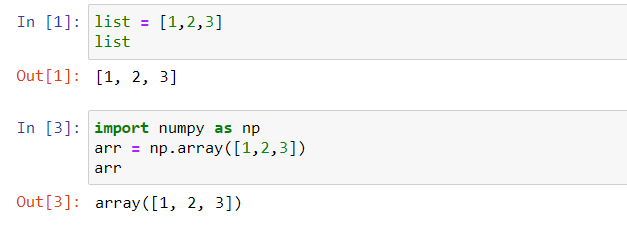Although, to make an array, you have to `import` the `numpy` library first. But still, it looks almost the same without an ‘array' text in front of them.

#### 2. Both data types are mutable

Both a list and array are mutable, it means that you can replace or change one of the data in a list or array.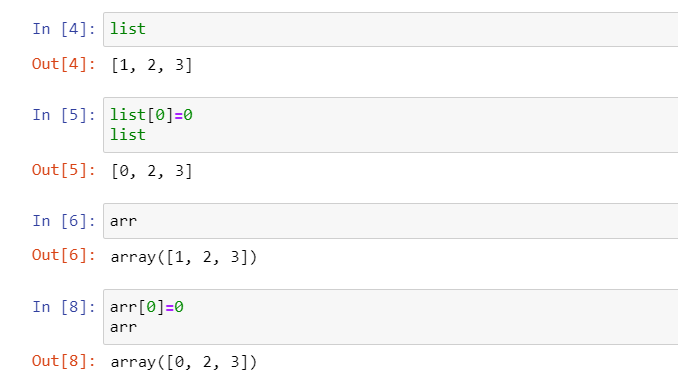This may also be the case difference between a list and a tuple where tuple is not mutable. But for a list and a set of arrays, you can change the data inside it.

#### 3. Both can be indexed and can be used for slicing operations

Yes. Both a list and an array can be indexed, it means that you can access the data from a list or an array through their indexes.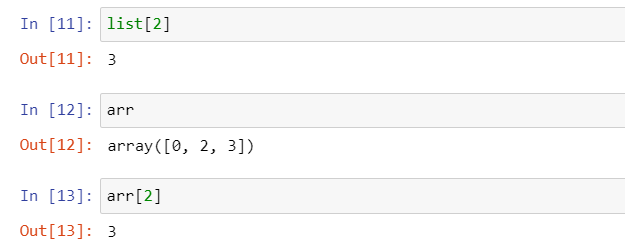Not only that, you can also use the slicing operations on both of them, it can come in handy when you're trying to filter out the data.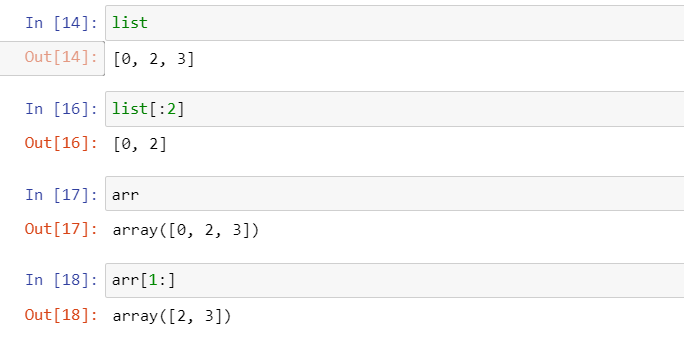After seeing the similarities between those two, we're back to the first questions.

### The differences between an array and a list?

#### 1. A list cannot directly handle a mathematical operations, while array can

This is one of the main differences between a list and array. While you can store an integer or float in a list, you can't really do mathematical operations in it.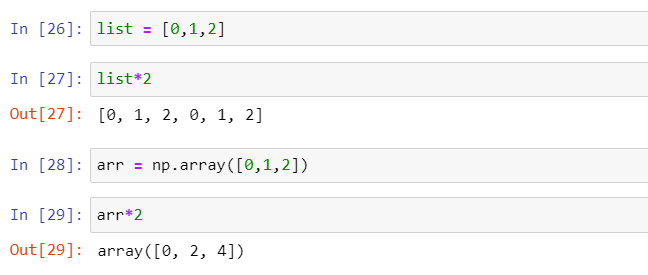See how using a * (multiply) in a list returns a repeated data in the list (while we meant to multiply all of the data in the list) and where using it on an array gives a correct or desired result.

This is why if you're dealing with lots of mathematical operations for your data, you should use an array. Although, you can also use a function in numpy to do a mathematical function to your list.

But, you still have to use the numpy libraries anyway, so why don't just use an array?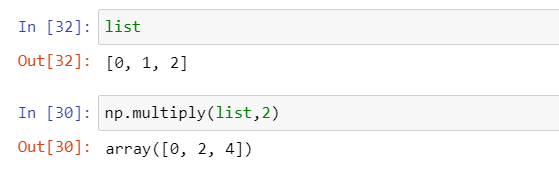#### 2. An array consumes less memory than a list

Okay, so from the first point you might say “I have the data in a form of list, as long as I can use mathematical operations in it, it doesn't matter. I don't have to change it into an array.”

Okay, but you might think twice after reading this.

Technically, a list can store different types of data while an array doesn't. This is one of the reasons why a list consumes more memory (it takes a lot of space to store different types of data, even though for this case you only use one type of data). This article explains it in a much more detailed way. So, if you're interested, you can read it here.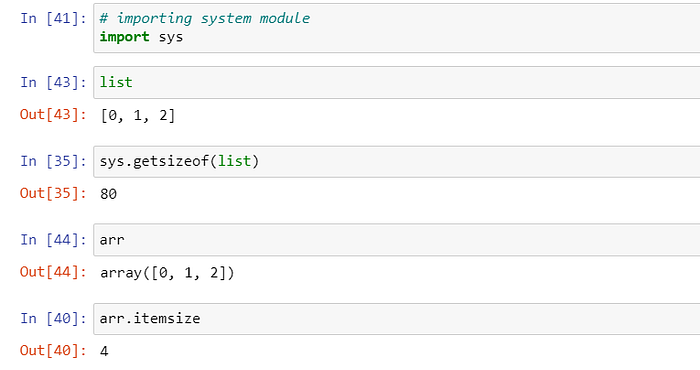Here you can see the difference between the memory it takes for one element in a list (80 bytes) vs a memory for one element in an array (4 bytes).

So, if you're dealing with a large data, using an array for your data is a good option.

#### 3. Using an array is faster than a list

Originally, Python is not designed for a numerical operations. In numpy, the tasks are broken into small segments for then processed in parallel. This what makes the operations much more faster using an array. Plus, an array takes less spaces than a list so it's much more faster.

#### 4. A list is easier to modify

So, if you're reading the first 3 point, you might say “Wow all signs points out that I should use an array, might as well use it and never use a list anyway.”

Well, this point might change your mind again. While an array has a lot of benefits, so does a list.

A list is easier to modify than an array does. Since a list store each element individually, it is easier to add and delete an element than an array does.

#### 5. A list can consist of different nested data size

While you can have a nested data with different size in a list, you can't do the same in an array. You have to have the same size (row and column) in an array, but you don't have to do that in a list.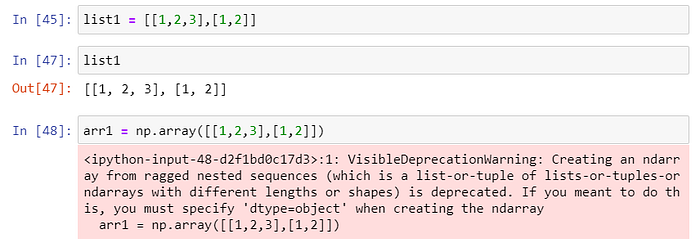#### 6. A list can store different data types

Another benefit of using a list is that you can use different data types store in a list, while you can't do that in an array. This what makes you think twice to use a list or an array if your data consist of different data types.

### Conclusion

Thank you for reading my post! I hope you have found this useful and now know more about the differences between both datatypes.